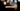# Leetcode | Solution of Decompress Run-Length Encoded List in JavaScript

April 6th, 2020
|

In this post, we will solve Decompress Run-Length Encoded List from leetcode and compute it's time and space complexities. Let's begin.

# Problem Statement

The question can be found at leetcode decompress run-length encoded list problem

The problem states that we are given an encoded array and we need to return the decoded array.

Let's take a look at the encoding. If the array is `[1,2,3,4]`, we have to interpret it in pairs. For each pair starting from `i = 0`, the first value is the frequency of the message and the second value is the actual value. So in our case, `1` here is the frequency of `2`. That means, `2` is present `1` time in the message. Similarly, `4` is present `3` times in the message.

# Solution

Here's the approach that we'll take

• We will declare an empty array.
• Next, we will loop over the array and for every pair, we will push the value in the array as many times as the frequency given in the pair
• At last, we return the array

We have discussed the approach, I urge you to go ahead on leetcode and give it another try.If you are here, it means something went wrong in implementation or you are just too lazy. In any case, let's see a simple implementation of the above logic.
``````var decompressRLElist = function (nums) {
const res = [];

for (let i = 0; i < nums.length; i += 2) {
for (let j = 0; j < nums[i]; j++) {
res.push(nums[i + 1])
}
}

return res;
};``````

The solution is very straight forward. Not much to discuss here.

Here are the stats on submission

``````Status: Accepted
Runtime: 116ms
Memory: 36.6MB``````

## Time and space complexity

### Time complexity

We have a nested loop here, so time complexity would be O(n2)

### Space complexity

We are using extra space for the array. So space complexity is linear, O(n).

# Summary

So, we solved the Decompress Run-Length Encoded List and calculated the time and space complexities.

I hope you enjoyed solving this question. This is it for this one, complete source code for this post can be found on my Github Repo. Will see you in the next one.